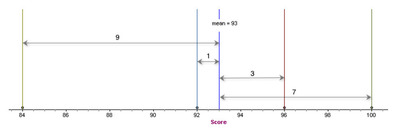# Measuring Variability in a Data Set

Alignments to Content Standards: S-ID.A.2 S-ID.A.3

Jim has taken 4 exams in his Statistics class.

 Exam Score 1 92 2 84 3 96 4 100

1. Both the MAD (mean absolute deviation) and the standard deviation are commonly used to measure variability in a data set. Explain how the way the standard deviation is calculated is different than the way the MAD is calculated.

2. Calculate the standard deviation of Jim’s scores and explain how this value represents the variability in his test scores.

3. Sally took the same exams and also had a mean score of 93. Both students’ scores are displayed in the dot plots below. Without calculating, determine if Sally’s standard deviation is larger than, smaller than or equal to the standard deviation for Jim’s scores. Explain your reasoning.4. Tom also took the same exams. His mean was 91 and his standard deviation was zero. What scores did Tom receive on his exams?

## IM Commentary

In high school, students build on their experience with the mean absolute deviation (MAD) in middle school. The standard deviation is introduced as a measure of variability, and students calculate and interpret the standard deviation in context. The purpose of this task is to develop students’ understanding of standard deviation (S-ID.2).

In the discussion of question 1, make sure that students can explain the differences in the way the standard deviation and the MAD are calculated (squaring instead of absolute value and division by n-1 instead of n).

Other discussion points include the following.

• Students are familiar with MAD (mean absolute difference) and should be able to discuss how the standard deviation is related to the MAD.

• Students should recognize which of the data points contribute the most to the size of the standard deviation.

• Students should recognize the purpose of squaring each deviation. Note that the sum of the deviations will always sum to zero.

• Note that the score of 84 on Jim’s first exam contributes the most to the size of the standard deviation and 92 contributes the least. These correspond to the scores that are furthest away from and closest to the mean respectively.

## Solution

1. The standard deviation is a measure of spread about the mean and is defined as

Standard Deviation = $\sqrt{{1 \over{n-1}} \sum {{(x - \bar{x})}^2}}$

MAD = ${1 \over{n}} \sum {{ |x - \bar{x}|}}$

While both measures rely on the deviations from the mean ($x - \bar{x}$), the MAD uses the absolute values of the deviations and the standard deviation uses the squares of the deviations. Both methods result in non-negative differences. The MAD is simply the mean of these nonnegative (absolute) deviations. The standard deviation is the square root of the sum of the squares of the deviations, divided by (n-1). This measure also results in a value that in some sense represents the “typical” difference between each data point and the mean.

2.  To calculate the standard deviation, we must first find the mean of the test scores. $\bar{x} = {{92+84+96+100} \over {4}} = {{372} \over {4}} = 93$. Then, we find the deviations from the mean, find the sum of the squares of the deviations, divide by one less than the number of scores and take the square root of the result.Exam Score $x- \bar{x}$ $(x - \bar{x})^2$ 1 92 92 - 93 = -1 1 2 84 84 - 93 = -9 81 3 96 96 - 93 = 3 9 4 100 100 - 93 = 7 49

$\sqrt{{1 \over{n-1}} {\sum{(x - \bar{x})}^2}} = \sqrt{{1 \over {3}}(1+81+9+49)}= \sqrt{{140} \over{3}}=\sqrt{46.67} = 6.83$.

This value is larger than the mean absolute difference between the mean and each test score, which is MAD = ${1 \over{n}} \sum{|x- \bar{x}|} = {1 \over{4}}(1+9+3+7)=5.$. Because the standard deviation is based on the squared deviations from the mean, it will be large when the values in a data set are spread out around the mean and small when the values are tightly clustered.

3.  Sally’s scores and Jim’s scored both have a mean score of 93. Since Sally’s scores are clustered more closely around 93 than Jim’s scores, Sally’s scores are less variable and the standard deviation of her scores will be smaller than the standard deviation of Jim’s scores.

4.  In order for the standard deviation to be zero, all scores must be the same. So Tom must have scored 91 on all four exams.